Smartick is a fun way to learn math!Mar23

# Identifying Flat Symmetrical Figures

In today’s post, I am going to present a series of exercises to practice how to identify flat symmetrical figures.

To begin, I recommend that if you don’t remember symmetry or the characteristics that make a figure symmetrical very well, take a look at this previous post. You’ll be able to review all of the most important aspects of symmetry:

Now that you are ready, we are going to practice with a few exercises.

#### Complete the Figure so That It Is Symmetrical

In order to complete these exercises, you need to choose the picture that is exactly the same as the picture shown, but in reverse, from the options below. This way, you practice the concept of the internal symmetry of an object.Solution: Image 1

#### If We Fold the Figure on the Green Line Will the Two Parts Match?

To complete this activity you must imagine that you fold the figure along the green line as if it were made of paper. Then, think about what would happen, would both parts overlap and match up with each other as one? Or would one side hang lower than the other?

By doing this activity mentally we are working on the concept of the internal axis of symmetry of a figure.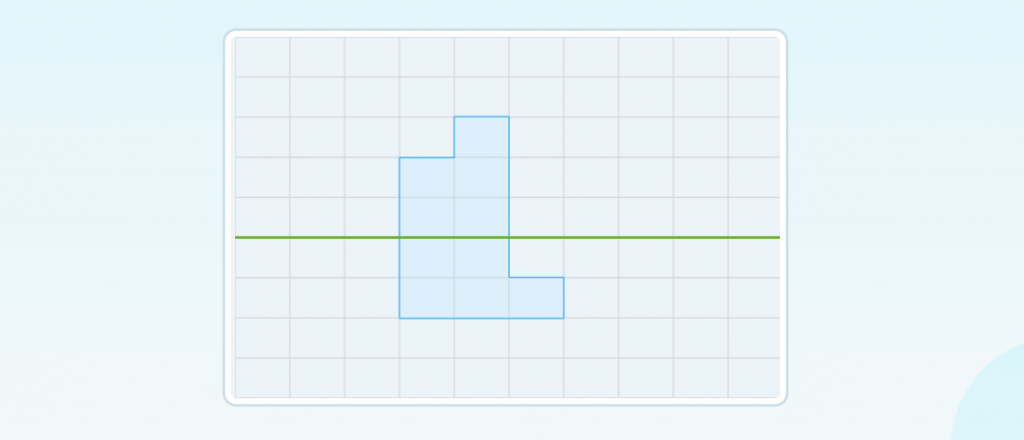Solution: They do not match up if we fold it.

#### Does the Green Line Divide the Figure into Two Symmetrical Parts?

For this activity, you need to identify if the two parts of the figure divided by the green line are the same but opposites.

By doing this, you work on the axis of symmetry horizontally.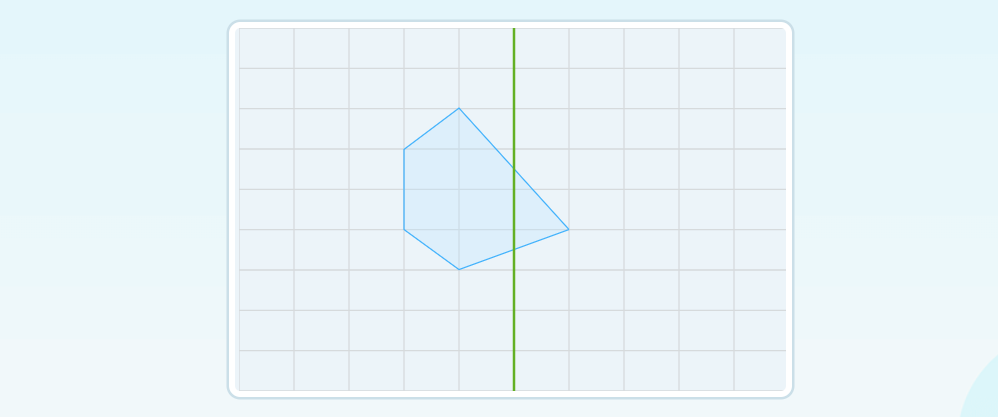Solution: The green line does not divide the figure into two symmetrical parts.

#### Is This Figure Symmetrical?

You just need to look closely at the shape of the figure in this activity and how it is arranged in relation to the green line.

With this activity, you will learn to recognize the indicators that tell you whether or not a figure is symmetrical.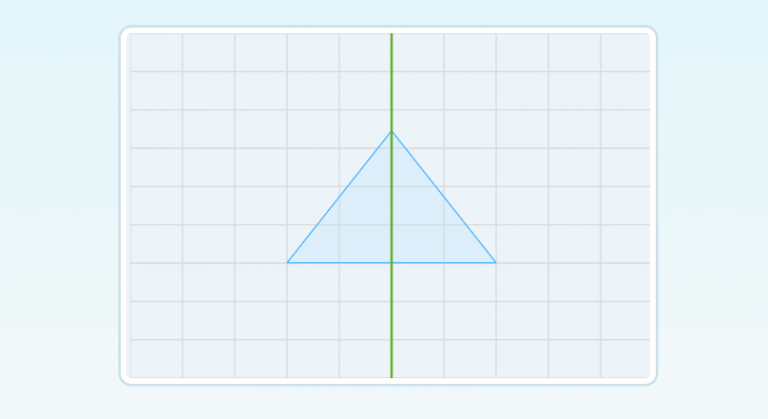Solution: Yes, it is symmetrical.

#### Is This the Axis of Symmetry of the Figure?

To solve this type of activity you need to look closely at the position of the axis with respect to the figure.

The objective is to learn that a figure can be symmetrical but cut in a place that is not its axis of symmetry.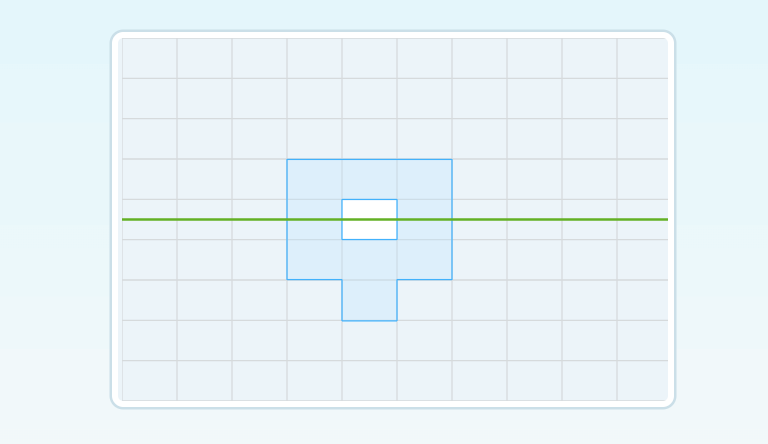Solution: No, this is not the axis of symmetry of the figure.

#### Which of the Following Figures Are Symmetrical?

For this last activity, you need to take everything that you have learned previously and put it into practice.

With this exercise, you learn to compare symmetrical and non-symmetrical figures.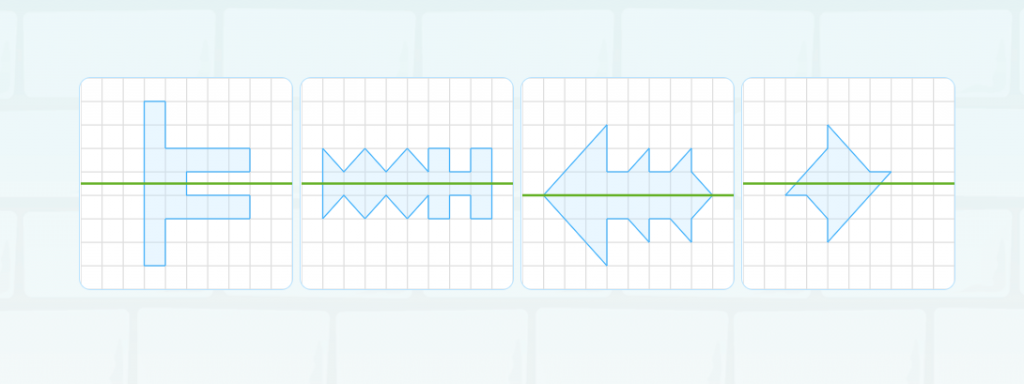Solution: Numbers 1, 2, and 3 are symmetrical.

With these types of activities, you have been able to learn all about the symmetry of a flat figure. I hope that you liked it!

If you would like to learn and practice more interesting activities like these, log onto Smartick and try our online learning method for free.